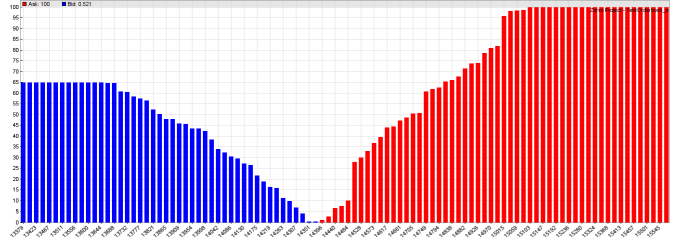# Market Depth / Order Flow Analysis

The following functions can be used for analyzing the order flow from the distribution of quotes and their volumes in the order book. The height of a bar in the image below is the cumulative volume of a price in the orders book, blue for bid and red for ask prices. The volume is expressed as a 0..1 percentage of the total ask or bid volume, whichever is higher. You can see that the situation in the diagram is dominated by offers (100% vs. 65%), which might indicate a further falling bitcoin price.BTC/USD June 2018, cumulative order volume distribution

## orderUpdate (string Name, int Handle): int

Loads the current list of quotes from the order book. In [Test] or [Train] mode the quotes are read out of a Name.t2 / Name_YYYY.t2 file containing historical order book content. In [Trade] mode the quotes are downloaded from the broker API. The quotes are stored in a dataset with the given Handle. Every record of the dataset contains 3 fields: the timestamp, the quoted price (negative for bid), and the quoted volume. The function sets the OrderRow variable to the row of the first quote in the dataset, and returns the number of quotes belonging to the current time and date.

## orderCVD (T2* Quotes, int N, var Distance): int

Generates a cumulative volume distribution of the price/volume pairs passed in the T2 list. The T2 struct is defined in trading.h and is identical to the quote format generated by orderUpdate, which must be called before. Distance is the maximum deviation of the highest ask or lowest bid from the center price. Returns the number of quotes within Distance.

## cpd(var Price): var

Returns the cumulative relative volumen of the given Price, in percent (0..100). 100 is equivalent to the total ask or bid volume, whichever is higher. Negative prices return the bid volume, positive prices the ask volume.

### Parameters:

 Name Name of the order book file, without the trailing ".t2" and without a possible year number. Handle Handle of the dataset to receive the order book data. Quotes List of quotes in T2 format. N Number of quotes. Distance Maximum order book range. Price Quote price.

### Remarks:

• The broker plugin must support the GET_BOOK command for order flow analysis. Zorro S is required.

### Example:

```void main()
{
StartDate = 20180110;
LookBack = 0;
BarPeriod = 10;
PlotScale = 10;
set(PLOTNOW);

assetList("AssetsCrypto");
asset("BTC/USD");

// load today's order book
int N = orderUpdate("BTCUSD",1);
T2* Quotes = dataStr(1,OrderRow,0);
printf("\nOrderbook: %i quotes",N);

var Distance = 0.05*price(); // +/- 5%
int N2 = orderCVD(Quotes,N,Distance);
printf(", %i in range",N2);
Distance *= 1.5;
var Price = priceClose() - Distance;
for(i = 0; i lt; 100; i++) {
Price += Distance/50;